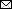### Changing Disease Course of Crimean-Congo Hemorrhagic Fever in Children, Turkey

Pembe Derin Oygar1, Sibel Laçinel Gürlevik, Erdal Sağ, Sare İlbay, Tekin Aksu, Osman Oğuz Demir, Yasemin Coşgun, Selin Aytaç Eyüpoğlu, Jale Karakaya, Şule Ünal Cangül, Ali Bülent Cengiz, and Yasemin ÖzsürekciAuthor affiliations: Hacettepe University, Ankara, Turkey (P.D. Oygar, S.L. Gürlevik, E. Sağ, T. Aksu, O.O. Demir, S.A. Eyüpoğlu, J. Karakaya, Ş.Ü. Cangül, A.B. Cengiz, Y. Özsürekci); İhsan Doğramacı Children’s Hospital, Ankara (P.D. Oygar, S.L. Gürlevik, T. Aksu, O.O. Demir, S.A. Eyüpoğlu, Ş.Ü. Cangül, A.B. Cengiz, Y. Özsürekci); Ankara Training and Research Hospital, Ankara (E. Sağ); Hacettepe University Rheumatology Translational Medicine Laboratories, Ankara (S. İlbay); General Directorate of Public Health, Ministry of Health, Microbiology Reference and Biological Materials Laboratory, Ankara (Y. Coşgun)

Main Article

#### Table 4

Variations in correlation of laboratory parameters with cytokines/chemokines for 12 pediatric case-patients with Crimean-Congo hemorrhagic fever, based on time interval, Turkey*†

Cytokine/chemokine Neutrophil count Platelet count Fibrinogen D-dimer a-PTT INR Ferritin LDH ALT TG
IFN-α
NA
NA
r2 = 0.726; p2 = 0.027
NA
NA
r1 = 0.720; p1 = 0.029
NA
NA
NA
r2 = 0.840; p2 = 0.018
IFN-γ
NA
NA
r2 = 0.770; p2 = 0.015
NA
NA
NA
r1 = 0.0857; p1 = 0.007
NA
NA
r2 = 0.863; p2 = 0.012
CXCL10
NA
NA
NA
r3 = 0.669; p3 = 0.049
r2 = 0.677; p2 = 0.045
NA
NA
NA
NA
r1 = −0.920; p1 = 0.009
CXCL11
NA
NA
NA
NA
NA
NA
NA
r3 = −0.716; p3 = 0.046
NA
r1 = −0.851; p1 = 0.032
MIP-3α
r1 = −0.783; p1 = 0.013
NA
NA
NA
NA
NA
r1 = 0.771; p1 = 0.025
r1 = 0.667; p1 = 0.05
NA
NA
IL-1β
NA
NA
NA
NA
NA
NA
NA
NA
NA
r2 = 0.883; p2 = 0.009
lL-10
NA
r3 = −0.748; p3 = 0.021
NA
NA
NA
NA
NA
NA
NA
r2 = 0.870; p2 = 0.011
IL-18
r1 = −0.721; p1 = 0.029
r1 = −0.762; p1 = 0.017;
r1 = −0.676; p1 = 0.046; r2 = 0.693; p2 = 0.038
NA
NA
NA
NA
r1 = 0.670; p1 = 0.048
r2 = 0.688; p2 = 0.049
NA
IL-33
NA
NA
r2 = 0.943; p2 = 0.001
NA
NA
NA
NA
NA
r2 = 0.739; p2 = 0.023
r2 = 0.851; p2 = 0.015
CCL17
NA
r1 = 0.823; p1 = 0.006; r2 = 0.851; p2 = 0.004
NA
NA
r1 = −0.733; p1 = 0.025
NA
NA
r3 = −0.907; p3 = 0.002
NA
NA
CCL11
NA
NA
NA
r2 = −0.758; p2 = 0.018; r3 = −0.668; p3 = 0.049
NA
NA
r2 = −0.681; p2 = 0.043
r2 = −0.669; p2 = 0.049; r3 = −0.895; p3 = 0.003
NA
NA
ENA78 NA NA NA NA NA NA NA NA NA NA

*First time interval, 68–72 h after baseline; second, 120–132 h; third, 156–180 h. CCL, CC chemokine ligand; CXCL, C-X-C motif chemokine ligand; ENA, epithelial neutrophil-activating; IL, interleukin; IFN, interferon; IP, IFN-γ-inducible protein; I-TAC, interferon-inducible T-cell alpha chemoattractant; RANTES, regulated upon activation, normal t cell expressed and presumably secreted; MIP, macrophage inflammatory proteins; NA, not applicable; TARC, hymus- and activation-regulated chemokine. †r1, correlation coefficient during the first time interval; p1, asymptotic significance (2-tailed) during the first time interval; r2, correlation coefficient during the second time interval; p2, asymptotic significance (2-tailed) during the second time interval; r3, correlation coefficient during the third time interval: p3, asymptotic significance (2-tailed) during the third time interval.

Main Article

1Current affiliation: Nicosia State Hospital, Burhan Nalbantoglu General Hospital, Nicosia, Cyprus.

Page created: December 07, 2022
Page updated: January 21, 2023
Page reviewed: January 21, 2023
The conclusions, findings, and opinions expressed by authors contributing to this journal do not necessarily reflect the official position of the U.S. Department of Health and Human Services, the Public Health Service, the Centers for Disease Control and Prevention, or the authors' affiliated institutions. Use of trade names is for identification only and does not imply endorsement by any of the groups named above.